﻿ MATS347 Composites Design and Manufacture
 Composites Design and Manufacture (Plymouth University teaching support materials)Young's moduli, Poisson's ratios, shear and bulk moduli LecturePowerPoint ReadingLists Reviewpapers SubjectIndexGo direct to:

 E Young's modulus

Hooke's Law  originally stated that a change in length was proportional to the load, but now it has been generalised to denote that the stress (σ) is proportional to the strain (ε) with the constant of proportionality known as Young's modulus (E), so that σ = Eε.  Traditional structural engineering materials are isotropic and hence the value of E is independent of direction.  However, the Young's modulus of a composite material is anisotropic (varies with direction) and can be estimated using the rule-of-mixtures :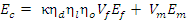Equation 1

where:

Ec = Young's modulus of the composite
Ef = Young's modulus of the fibre
Em = Young's modulus of the matrix
Vf = fibre volume fraction
Vm = matrix volume fraction (1-Vf-Vv)
Vv = void volume fraction
κ = fibre area correction factor (set at unity for circular cross-section fibres)
ηd = fibre diameter distribution factor (set at unity for man-made fibres)
ηl = fibre length distribution factor
ηo = fibre orientation distribution factor

For a unidirectional continuous fibre composite aligned with the stress, the physical assumption underlying the equation above is compatibility of strain between the fibre and the matrix resulting in the constituent phases carrying load in proportion to their respective volume fractions and moduli.  Further, the above equation has a number of underlying assumptions [3-6]:

• each composite ply is orthotropic, macroscopically homogeneous and linearly elastic
• the fibres are homogeneous and linearly elastic
• if the fibres are orthotropic, then a more general form of the equation may be required.
• the matrix is homogeneous and linearly elastic
• both the fibre and the matrix are free of voids
• each lamina has uniform thickness across the layer
• the interface is completely bonded with "no slip" between adjacent layers
• there is no transition region (no third party material) between the matrix and the reinforcement
• all displacements and strains are small
• there is equal strain in the fibre and the matrix
• the transverse shear stresses on the upper and lower surfaces are zero 
• the laminate deforms in accordance with the Kirchoff-Love plate theory:
• all normals to the mid-surface remain straight and normal after deformation
• the thickness is unchanged after deformation
• Poisson's strains are neglected (the Poisson's ratio of the fibre and the matrix are the same)
• if the Poisson's ratios differ, then the neglected term has been shown to be of the order of the square of the difference between the two values 
• the assumptions described below for fibre length distribution factor and fibre orientation distribution factor also apply.

The rule of mixtures can be used for other "elastic" properties (e.g. density, Poisson's ratio, coefficient of thermal expansion and hygrothermal properties).

The fibre length distribution factor (FLDF) assumes that:

• the matrix and fibre remain elastic and the interface bond is perfect,
• the shear stress at the fibre ends is maximum and falls to zero after half the "critical length", and
• the tensile stress at the fibre ends is zero and rises to a maximum after half the "critical length", as shown in Figure 1.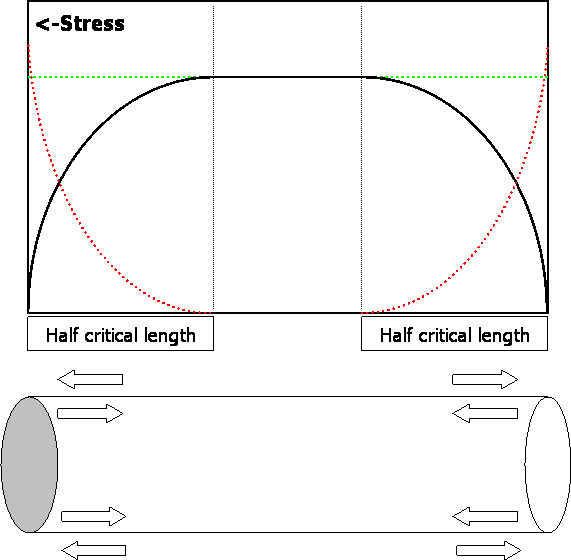Figure 1: Diagrammatic representation of the stress distribution in a short fibre,
with shear stress at the fibre matrix interface shown in red, tensile stress in black and peak stress (Efε) shown in green.

FLDF can be calculated using the Cox equation :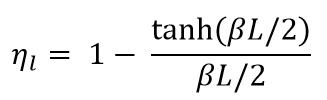Equation 2 

where: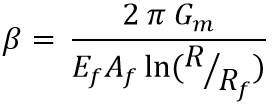Equation 3  and: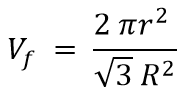Equation 4 

and where Gm is the shear modulus of the matrix, L is the fibre length, Af is the cross sectional area of the fibre, Rf is the radius of the fibre and R is the mean separation of the fibres.  If the fibre is shorter than the critical length (lc), it will never carry a load high enough to cause fibre fracture.  The composite will instead fail in shear, either at the fibre/matrix interface or in the matrix itself.  Alternative Equations for the prediction of β have been proposed by Nayfeh  and McCartney .  Nairn  has extended the shear-lag analysis from concentric cylinders to a generalized form (with an new βcor as Equation 44 in his paper) with transverse variations of shear stress described by arbitrary shape functions. The new analysis permits modelling of imperfect interfaces both between concentric cylinders  and within multilayered structures . Modification of the prior shape functions permits extended shear-lag analysis to work for any fibre volume fraction (previous models were unacceptable at low fibre volume fraction). The full shear-lag analysis can model stress transfer for both isotropic and anisotropic fibres.

The critical length (when there is no debonding) is given by the expression: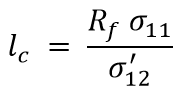Equation 5 

where R is the fibre radius, σ11 is the tensile stress in the fibre and σ'12 is the shear strength (of the interface or of the matrix as appropriate).

and the fibre orientation distribution factor can be calculated using the Krenchel equation :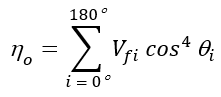Equation 6 

For a unidirectional ply, the rule-of-mixtures does assume that:

• the fibres are continuous with uniform cross-section and lie parallel to each other.
• there is perfect bonding between the fibre and the matrix and hence Cox shear-lag theory applies.
• a longitudinal load produces equal strain in both the fibre and the matrix.

and typical values used in the equation would be:

Ef = 70 GPa (glass), 140 GPa (aramid) or 210 GPa (carbon)
Em = 1-3 GPa (polymers) or 70 GPa (aluminium)
Vf = 0.1-0.3 (random), 0.3-0.6 (woven) or 0.5-0.8 (unidirectional)
ηl = 0 (if significantly less than the critical length) varying up to 1 (continuous fibres)
• ηo = 0.0 for unidirectional perpendicular to the fibres
• ηo = 1/4 for biaxial on the bias angle (at ±45º to the fibres)
• ηo = 3/8 for random in-plane
• ηo = 1/2 for biaxial parallel to the fibres
• ηo = 1.0 for unidirectional parallel to the fibres

For a continuous fibre carbon/resin composite (Ef = 210 GPa, Em = 3 GPa with Vf = 70% for unidirectional or Vf = 50% for bidirectional), Figure 2 illustrates how the Young's modulus calculated from the rule-of-mixtures varies with change in the angle between the applied stress and the fibre direction: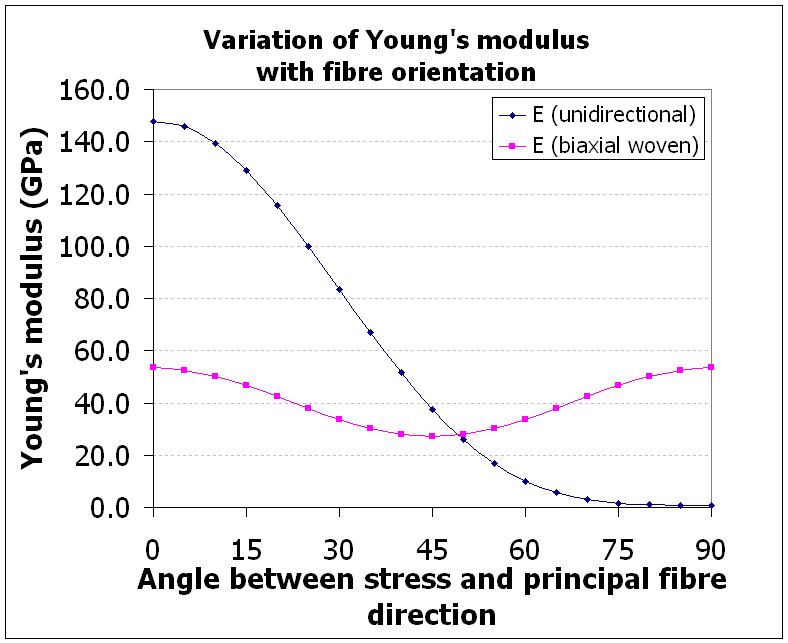Figure 2:  Variation of Young's modulus with fibre orientation

The materials data above is representative and should not be used for 'design' purposes.

The Voigt (1887)  constant strain and Reuss (1929)  constant stress models for load sharing in materials can be applied to composites.  One source [ NB: I cannot currently trace where I found the transition point at 0.5% strain :-( ] states that:
The Reuss model suggests that up to 0.5% strain, there is equal stress in both the fibres and the matrix.  The Reuss model for equal stress in the context of transverse stiffness is described in Hull and Clyne [18 page 63].
The Voigt model suggests that above 0.5% strain, there are equal increases in strain in both the fibres and the matrix.

 ν Poisson's ratio

Poisson's ratio is denoted by the Greek letter nu: ν.  It has a value determined by:

ν = -(strain normal to the applied stress)/(strain parallel to the applied stress)

Tensile deformation is taken as positive and compressive deformation is taken as negative. The minus sign in the definition of Poisson's ratio normally results in positive values of Poisson's ratio and a thermodynamic constraint which restricts the values to -1 < ν < 1/2.

For an orthotropic composite, there may be a different Poisson's ratio associated with each plane.  Maxwell’s reciprocal theorem  states that two strains must be equal if the two stresses are of equal magnitude and sense.  It is implicit in the symmetry of the stiffness/compliance matrices for a square symmetric material (E2 = E3) that ν12E2 = ν21E1, and hence ν12 = ν13, ν21 = ν31 and ν23 = ν32 Lemprière  generalised the above thermodynamic constraint for the case of orthotropic materials where both the stiffness and compliance matrices are positive-definite (i.e. E1, E2, E3, G23, G13, G12 > 0) to yield the following results:

(1-ν23ν32), (1-ν13ν31), (1-ν12ν21), (1-ν12ν2113ν3123ν32-2ν21ν32ν13) > 0

and thus

νij ≤ (Ei/Ej)1/2 and ν21ν23ν13 < 1/2.

Craig and Summerscales  measured the Poisson's ratios in all three planes for two glass-fibre laminates

C1: 13 layers of Fothergill and Harvey Y119 unidirectional rovings with 25% fibre volume fraction
(based on fabric areal weight = 640 gsm, fibre relative density = 2.56, panel thickness = 11 mm) [22a]
A2: 12 layers of TBA 830 gsm ECK25 woven rovings in Crystic 625TV resin
(based on fabric areal weight = 830 gsm [22b/c], fibre relative density = 2.56, panel thickness unknown)

and confirmed that the Lemprière criteria were valid for both materials (Table 1):

Table 1: Poisson's ratios and elastic properties for glass fibre laminates .
 Unidirectional Unidirectional Woven Woven Panel C1: ν, E or G Panel C1 √Ei/Ej Panel A2: ν, E or G Panel A2 √Ei/Ej ν12 0.308 1.606 0.140 0.942 ν21 0.123 0.623 0.109 1.061 ν13 0.354 1.687 0.408 1.285 ν31 0.124 0.593 0.247 0.778 ν23 0.417 1.051 0.380 1.364 ν32 0.414 0.952 0.297 0.733 E1 (GPa) 20.3 15.5 E2 (GPa) 7.9 17.5 E3 (GPa) 7.1 9.4 G12 (GPa) 3.45 3.0

If one Poisson's ratio in an orthotropic material is negative, then no restriction is placed on the other two values.  Dickerson and Di Martino  published data for cross-plied boron/epoxy composites in which the Poisson's ratios range from 0.024 to 0.878 in the orthotropic case and from -0.414 to 1.97 for a ±25º laminate.

Materials and structures with negative Poisson's ratios do exist and are termed auxetic.  The state-ot-the-art of auxetics research in the UK was reviewed by KTN in 2016 .  A variety of often re-entrant or chiral structures (Figure 3) achieve this effect, as in the animation below from Rod Lakes (University of Wisconsin) webpage on Negative Poisson's ratio materials: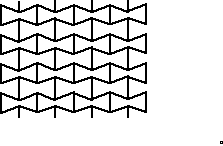............Figure 3:  Re-entrant (left) and chiral (right) auxetic (negative Poisson's ratio) structures (from Rod Lakes webpage)

Cork oak bark at high compressive strains has a negative Poisson's ratio under non-radial stresses, but has a positive Poisson's ratio when compressed in the radial direction .

 G Shear modulus

The inter-relationship of the elastic constants for isotropic materials is given by:

 G = E/2(1+ν) Equation 7  K= E/3(1-2ν) Equation 8 

where E is the Young’s modulus,  G is the shear modulus, K is the bulk modulus and ν is Poisson’s ratio.

For orthotropic materials within a single plane, Huber  proposed that the shear modulus would be predicted by: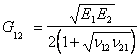Equation 9 [26, 27]

although his original work was for reinforced concrete slabs.  Panc  used theoretical considerations to show that the expression may be used as an approximation.  Cheng and He  and Bert et al  have considered the use of this Equation in the context of fibre-reinforced composites and conclude that although the relation is inaccurate, and can lead to large errors in the shear modulus G, the use of the relation in the governing differential equations does still yield very accurate final solutions.

Craig and Summerscales  used Huber’s equation to validate the elastic constants measured in the plane of the reinforcement for both unidirectional and woven fibreglass panels and presented data for the Poisson's ratios in all three orthogonal planes (Table 1).  For an orthotropic composite, the shear modulus in any plane will change as the fibre axes are rotated relative to the applied biaxial stress.  The optimum correlation of theory and experiment was obtained when Huber's equation was used to obtain the shear modulus G12(45°) rather than GLT measured when the sample is rotated by 45°.

Khashaba  used a modified Iosipescu test fixture to investigate the in-plane shear properties of cross-plied E-glass/epoxy composite laminates with different off-axis angles.  The maximum in-plane shear modulus (plus maximum Poisson's ratio and minimum Young's modulus) was found for specimens with [+45°/−45°]2s stacking sequence .  The minimum in-plane shear strength was found in specimens with [0°/90°]2s and [90°/0°]2s stacking sequences.  The experimental results agreed very well with the predicted in-plane shear moduli in Table 2. Orthotropic shear moduli for carbon fibre composites are given in Table 3.

Table 2:. The predicted elastic properties of an angle-ply E-glass/epoxy laminate with different off-axis angles (from Khashaba ).
 Off-axis angle Stacking sequence Exx=Eyy (GPa) νxy (GPa) Gxy (GPa) LY138-1/HY 5138 epoxy resin N/A 3.2 0.36 1.18 17 μm 1.15 g/m E-glass roving N/A 72.4 0.22 30.13 0° [0°/90°]2s 21.0 0.129 2.71 15° [+15°/-75°]2s 15.6 0.352 3.29 30° [+30°/-60°]2s 10.3 0.571 5.79 45° [+45°/-45°]2s 8.85 0.633 9.30 60° [+60°/-30°]2s 10.3 0.571 5.79 75° [+75°/-15°]2s 15.6 0.352 3.29 90° [90°/0°]2s 21.0 0.129 2.71

Table 3: In-plane shear moduli for a 32-ply ACG T700/LTM45 prepregs carbon/epoxy laminates using the Iosipescu shear method .
 Laminate G12 (GPa) G13 (GPa) G23 (GPa) unidirectional 4.6 ± 0.2 5.2 ± 0.3 3.0 ± 0.4 [0°4/90°4]2s 4.7 ± 0.14 4.5 ± 0.3 4.3 ± 0.05 [±45°/90°/0°]4s 17.9 ± 0.3 3.9 ± 0.2 3.9 + 0.2

 K Bulk modulus

By extension of Equation 8, Summerscales  proposed that the bulk modulus for a square symmetric material could be predicted from the following equation: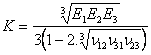Equation 10 

This equation reduces to the isotropic form when E1 = E2 = E3 and ν12 = ν21 = ν23.

Table 4 gives values for Young’s moduli E, Poisson’s ratios ν and bulk moduli Kref  (all moduli in GPa) for various materials.  The abbreviation (iso) is used to indicate isotropic properties.  The unidirectional carbon fibre composites in the Table are square symmetric.  Equations 9 and 10 have been used to predict the bulk moduli Kcalc for the isotropic and square symmetric materials respectively.

Table 4: Elastic constants for a variety of materials, based on data from  and the indicated references.
 Material E1 E2 E3 ν12 ν21 ν23 Kref Kcalc Ref. Epoxy resin 2.4 (iso) (iso) 0.34 (iso) (iso) - 2.5  Crown glass 71.3 (iso) (iso) 0.22 (iso) (iso) 41.2 42.4  Heavy flint glass 80.1 (iso) (iso) 0.27 (iso) (iso) 57.6 58.0  Graphite - - - - - - 33.0 - Carbon fibre/epoxy composites High-modulus (HM) parallel 287 7.75 7.75 0.3 0.01 0.55 - 11.3  High-strength (HS) perpendicular 177 11.7 11.7 0.33 0.02 0.47 - 13.6  High-strength (HS) parallel 172 11.6 11.6 0.36 0.024 0.48 - 14.0  Carbon fibres Tenax HTA5131 carbon fibre 238 28 28 - - - - - 

Using the mercury porosimetry technique, Matthews and Ridgway  have estimated the bulk modulus of woven Tenax HTA carbon fibre Injectex and twill fabrics (Brochier SA, Dagneux - France) at 20 GPa and 7 GPa respectively.  Both the Injectex and twill fabrics have the same fabric areal weight (290 gm-2) and are woven with fibres from the same batch.

Using the rule-of-mixtures, the unidirectional HS fibre composites might be assumed from the axial modulus to be ~70-75% fibre by volume of 238 GPa fibre.  This would suggest a contribution of ~0.75 GPa from the resin to the bulk modulus, leaving a fibre bulk modulus of ~13 GPa (average of parallel and perpendicular composite moduli minus resin contribution).  Note that this value lies between those estimated by Matthews and Ridgway.

The discrepancy between the predicted and measured bulk moduli for the carbon fibres may be due to any or all of the following assumptions:

• the woven fabric has a unit cell size of a similar magnitude to the dimensions of the porosimeter cell.  The limited sample of the cloth tested may not be representative of the material.
• the Injectex fabric does have a small proportion of polymer fibre to promote flow enhancement by restricting the collapse of the tow to the normal lenticular cross-section.
• the estimation of bulk modulus from the porosimeter data involves an element of interpretation.
• the difficulty of making accurate measurements of fibre Poisson’s ratios, especially in the transverse direction, and the implications of this for the prediction of the bulk moduli.
• the absence of a coherent data set of engineering properties for the specific fibres used.

The proposed equation for the prediction of the bulk modulus of anisotropic fibres gives values which are of the same order of magnitude as estimates from experimentally determined values.

Anisotropic elasticity theory

For information on implementing the above in tensor/matrix form, see the anisotropic elasticity theory page.

Stephen Grove: Rules of mixture for elastic properties (University of Plymouth)
Polymer Composites: layer property prediction (AEA Technology Materials Solutions)

References

1. JC Anderson, KD Leaver, JM Alexander and RD Rawlings, Materials Science, second edition, Thomas Nelson & Sons, Sunbury-on-Thames, 1974.  ISBN 0-17-771015-2.
2. AS Virk, W Hall and J Summerscales, Modulus and strength prediction for natural fibre composites, Materials Science and Technology, 2012, 28(7), 864-871.
3. A Brent Strong, Fundamentals of Composites Manufacturing: materials, methods and applications, Society for Manufacturing Engineers, Dearbon MI, 1989.  ISBN 0-87263-358-6. second edition, 2008. ISBN 978-0-87263-854-9.  PU CSH Library
4. RF Gibson, Principles of Composite Material Mechanics, McGraw-Hill, 1994. ISBN 0-07-023451-5. Third edition, 2012. ISBN 978-1-4398-5005-3.  PU CSH Library.
5. JN Reddy, Mechanics of laminated plates and shells: theory and analysis - second edition, CRC Press, Boca Raton FL, 2003.  ISBN 978-0-84931-592-3.
6. PM Mohite, Introduction to classical plate theory, National Programme on Technology Enhanced Learning ~ India, 2012, accessed 21 December 2016.
7. C Basdogan, Transverse Shear Stresses in Beams, MDesign course notes, Koç University, no date, accessed 21 December 2016.
8. HL Cox, The elasticity and strength of paper and other fibrous materials, British Journal of Applied Physics, 1952, 3(3), 72-79.
9. MJ Folkes, Short Fibre Reinforced Thermoplastics, Research Studies Press, Letchworth, 1982.  ISBN 0-471-10209-1.
10. AH Nayfeh, Thermomechanically induced interfacial stresses in fibrous composites, Fibre Science and Technology, July 1977, 10(3), 195-209.
11. LN McCartney, Analytical models of stress transfer in unidirectional composites and cross-ply laminates, and their application to the prediction of matrix/transverse cracking, in JN Reddy and KL Reifsnider (editors), Proceedings of the IUTAM SYmposium, Blacksburg VA, 1992, 251-282.
12. JA Nairn, Generalized shear-lag analysis including imperfect interfaces, Advanced Composite Letters, 2004, 13(6), 263-274. Author's proof.
13. JA Nairn, On the use of shear-lag methods for analysis of stress transfer in unidirectional composites, Mechanics of Materials, 1997, 26(2), 63–80.
14. JA Nairn and DA Mendels, On the use of planar shear-lag methods for stress-transfer analysis of multilayered composites, Mechanics of Materials, 2001, 33(6), 335-362.
15. H Krenchel, Fibre Reinforcement, Akademisk Forlag, Copenhagen, 1964.
16. W Voigt, Theoretische Studien über die Elasticitätsverhältnisse der Krystalle I: Ableitung der Grundgleichen aus der Annahme mit Polarität begabter Moleküle, Abhandlungen der Königlichen Gesellschaft der Wissenschaften in Göttingen, Mathematischen Classe, 1887, 34(1), 3–52.
17. A Reuss, Berechnung der Fließgrenze von Mischkristallen auf grund der Plastizitätsbedingung für Einkristalle, Journal of Applied Mathematics and Mechanics, 1929, 9(1), 49–58.
18. D Hull and TW Clyne, An Introduction to Composite Materials: second edition, Cambridge University Press, August 1996. ISBN 0-521-38855-4.  PU CSH Library.
19. NJ Hoff, The Analysis of Structures, John Wiley, New York, 1956, p.373.
20. BM Lemprière, Poisson's ratio in orthotropic materials, AIAA Journal, 1968, 6(11), 2226-2227.
21. PD Craig and J Summerscales, Poisson’s ratios in glass fibre reinforced plastics, Composite Structures, 1988, 9(3), 173-188.
22. (a) SJ Thompson, RT Hartshorn and J Summerscales, Strain gauges on glass fibre reinforced polyester laminates, In IH Marshall (editor) Composite Structures 3 (Proc. 3rd Int.Conf.Composite Structures), Elsevier Applied Science Publishers, Barking, 1985, pp 748-759.
(b) RC Wyatt, LS Norwood, and MG Phillips, The stress-rupture behavious of GRP laminates in aqueous environments, In IH Marshall (editor) Composite Structures 1 (Proc. 1st Int.Conf.Composite Structures), Elsevier Applied Science Publishers, Barking, 1981,
(c) MF Crowther, MG Phillips and RC Wyatt, Design stress considerations for GRP components of power station cooling-water systems, In IH Marshall (editor) Composite Structures 3 (Proc. 3rd Int.Conf.Composite Structures), Elsevier Applied Science Publishers, Barking, 1985, 540-561.
23. EO Dickerson and B di Martino, Off-axis strength and testing of filamentary materials for aircraft application, 10th National Symposium, SAMPE, San Diego, 9-11 November 1966, H23-H50.
24. S Morris, A State of the Art Review of Smart Materials: a review of auxetics in the UK, Knowledge Transfer Network, March 2016.
25. SP Silva, MA Sabino, EM Fernandes, VM Correlo, LF Boesel and RL Reis, Cork: properties, capabilities and applications, International Materials Reviews, 01 December 2005, 50(6), 345-365.
26. MT Huber, Die Theorie der Kreuzweise bewehrten Eisenbetonplatten nebst Anwendungen auf mehrere bautechnisch wichtige Aufgaben uber Rechteckplatten (The theory of crosswise reinforced ferroconcrete slabs and its application to various important constructional problems involving rectangular slabs), Der Bauingenieur, 1923, 4(12), 354-360 and 4(13), 392-395.
27. V Panc, Theories of elastic plates, Noordhoff International Publishing, Netherlands, 1975.
28. S Cheng and FB He, Theory of orthotropic and composite cylindrical shells, accurate and simple fourth-order governing equations, ASME Journal of Applied Mechanics, December 1984, 51(4), 736-744.
29. CW Bert, Discussion and author's closure: Theory of orthotropic and composite cylindrical shells, accurate and simple fourth-order governing equations, Transactions of the ASME, December 1985, 52(4), 982-983.
30. UA Khashaba, In-plane shear properties of cross-ply composite laminates with different off-axis angles, Composite Structures, August 2004, 65(2), 167–177.
31. G Zhou, ER Green and C Morrison, In-plane and interlaminar shear properties of carbon/epoxy laminates, Composites Science and Technology, 1995, 55(2), 187–193.
32. J Summerscales, The bulk modulus of carbon fibres, Journal of Materials Science Letters, 2000, 19(1), 15-16.
33. GWC Kaye and TH Laby, Tables of Physical and Chemical Constants, 16th edition, Longman, Harlow, 1995. ISBN 0-582-22629-5.
34. NG McCrum, CP Buckley and CB Bucknall, Principles of Polymer Engineering - second edition, Oxford Science Publications, Oxford, 1997.  ISBN 0-19-856526-7.  PU CSH Library.
35. G Bradfield, Use in industry of elasticity with the help of mechanical vibrations, Note on Applied Science 30, HMSO, London, 1964.
36. BE Read and GD Dean, The Determination of Dynamic Properties of Polymers and Composites, Adam Hilger Limited, Bristol, 1978.  ISBN 0-85274-363-7.
37. JR Lowe (Tenax Fibers GmbH, Wuppertal), private communication, 2 June 1999.
38. GP Matthews and CJ Ridgway, Simulation of resin intrusion during injection moulding, Proceedings of the 5th International Conference on Flow Processes in Composite Materials, University of Plymouth, 12-14 July 1999, ACMC, Plymouth, pp 91-97.  ISBN 1-870918-01-0.